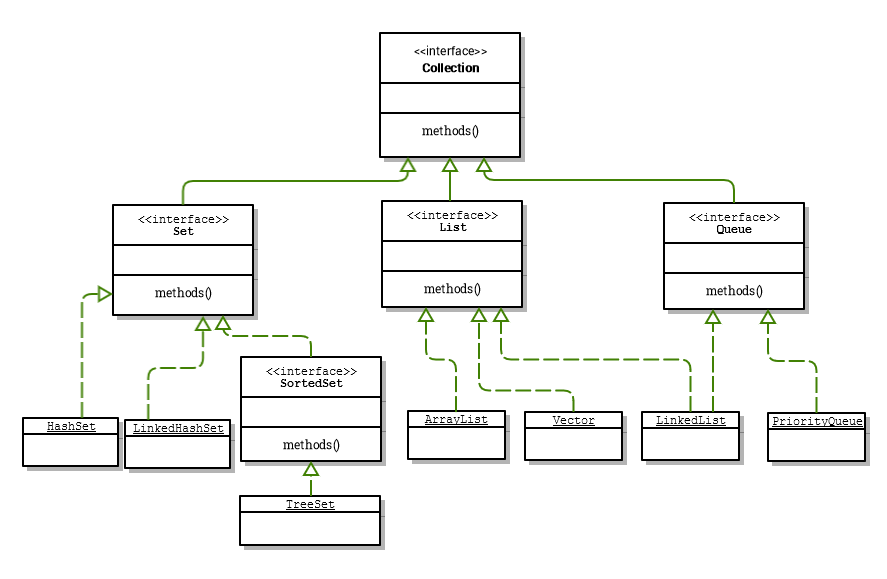## Difference between HashSet vs. TreeSet and LinkedHashSet ?

A Set should not contain duplicate elements. That is one of the major reasons to use a set. There are 3 implementations of Set

• HashSet
• TreeSet

Let us understand how to choose the specific Set implementation based on your requirement. In brief :

• If you need a fast set, you should use HashSet.
• If you need a sorted set, then use TreeSet.
• If you need a set that can store the insertion order of the elements then LinkedHashSet should be used.

Set Interface: Set interface extends Collection interface. In a set, no duplicate elements are allowed. Every element in a set must be unique. You can simply add elements to a set, and duplicates will be removed automatically.HashSet vs. TreeSet vs. LinkedHashSet Implementation

• `HashSet `is implemented using a hash table. Elements are not ordered. The add, remove, and contains methods have constant time complexity O(1).
• `TreeSet` is implemented using a tree structure (red-black tree). The elements in a set are sorted, but the add, remove, and contains methods have time complexity of O(log (n)). It offers several methods to deal with the ordered set like first(), last(), headSet(), tailSet(), etc.
• `LinkedHashSet `is between HashSet and TreeSet. It is implemented as a hash table with a linked list running behind it, so it provides the order of insertion. The time complexity of basic methods is O(1).

TreeSet Example :

```public static void main(String[] args) {
TreeSet treeSet = new TreeSet();

Iterator iterator = treeSet.iterator();
System.out.print("Tree set data in sorted order: ");
while (iterator.hasNext()) {
System.out.print(iterator.next() + " ");
}
}```

Output is sorted as follows:

``Tree set data in sorted order: 1 2 50 100 ``

Now let's define a Pizza class :

```class Pizza {

protected String type;
private int price;

public Pizza(String type, int price) {
super();
this.type = type;
this.price = price;
}

@Override
public String toString() {
return "Pizza [type=" + type + ", price=" + price + "]";
}
}```

Let's add some Pizzas to TreeSet. Here our expectation is that all the added pizzas will be printed in sorted order based on the price of the Pizza.

```package com.tuturself.basic;

import java.util.Iterator;
import java.util.TreeSet;

class Pizza {

protected String type;
private int price;

public Pizza(String type, int price) {
super();
this.type = type;
this.price = price;
}

@Override
public String toString() {
return "Pizza [type=" + type + ", price=" + price + "]";
}
}

public class TestTreeSet {
public static void main(String[] args) {
TreeSet treeSet = new TreeSet();

Iterator iterator = treeSet.iterator();

while (iterator.hasNext()) {
System.out.print(iterator.next() + " ");
}
}
}```

Compile ok, but run-time error occurs:

``````Exception in thread "main" java.lang.ClassCastException: com.tuturself.basic.Pizza cannot be cast to java.lang.Comparable
at java.util.TreeMap.compare(Unknown Source)
at java.util.TreeMap.put(Unknown Source)
at com.tuturself.basic.TestTreeSet.main(TestTreeSet.java:26)``````

Because TreeSet is sorted, the Pizza class needs to implement` java.lang.Comparable's compareTo() `method like the following :

```package com.tuturself.basic;

import java.util.Iterator;
import java.util.TreeSet;

class Pizza implements Comparable{

protected String type;
private int price;

public Pizza(String type, int price) {
super();
this.type = type;
this.price = price;
}

@Override
public int compareTo(Pizza p) {
return this.price - p.price;
}

@Override
public String toString() {
return "Pizza [type=" + type + ", price=" + price + "]";
}
}

public class TestTreeSet {
public static void main(String[] args) {
TreeSet treeSet = new TreeSet();

Iterator iterator = treeSet.iterator();

while (iterator.hasNext()) {
System.out.println(iterator.next() + " ");
}
}
}```

Now the output is sorted based on the price of the Pizzas:

``````Pizza [type=VEG, price=20]
Pizza [type=CHICKEN, price=40]
Pizza [type=CHICKEN With Topping, price=60] ``````

HashSet Example : In case of HashSet we cannot add any duplicate elements. HashSet does not retain the insertion order. The iteration order can be different than the insertion order. If we want to add custom objects like Pizza in HashSet and still wants to disallow adding duplicate elements, then we must implement hashCode() and equals() method properly for the custom Object.  Click here to read more about hashcode() and equals().

```HashSet hashSet = new HashSet();
Iterator iterator = hashSet.iterator();
while (iterator.hasNext()) {
System.out.print(iterator.next() + " ");
}

Output:
Pizza [type=CHICKEN With Topping, price=60]
Pizza [type=VEG, price=20]
Pizza [type=CHICKEN, price=40] ```

Note the order is not certain.

LinkedHashSet Example : LinkedHashSet maintains a linked list of the entries in the set, in the order in which they were inserted. This allows to retain the insertion-order while iterating over the set. That is, when iterating over a LinkedHashSet using an iterator, the elements will be returned in the order in which they were inserted. The hash code of the element is used as the index at which the data associated with the key is stored. The transformation of the key into its hash code is performed automatically.

```public class LinkedHashSetExample {
public static void main(String args[]) {

``[T, U, t, u, R, SELF]``
Remember Set does not allow duplicate values. So if we use uppercase T for both the cases then the second one will be identified as duplicate one and will not be added to the set